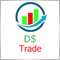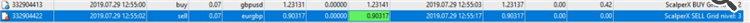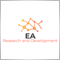# Incorrect TP value1228

Hello Developers,

I need your help to solve a peculiar case.
My problem happens when sending TP and SL for orders.
EA seeks TP of only 10 points. However when opening orders this distance is not being respected. For example, if I open a buy order by placing a 10-point TP, there are times when the order closes at 0 profit or sometimes even in lose trade.
am using for BUY orders the sum of TP along with Ask price and SELL orders with Bid price

I don't know if I'm handling orders in the right way. Can anyone please analyze my code and help me solve this problem?

In the following image the EA had a TP of 10 points or 1 pip, in the first order respect the values, but in the second order opened with the same entry price.I also attached the original EA

Thank you

```//+------------------------------------------------------------------+
//|                                                      ProjectName |
//|                                       http://www.companyname.net |
//+------------------------------------------------------------------+
input int magic=2019; //magic
input int TP=5; //Take Profit
input int SL=800; //Stop Loss
input bool useLotPerEquity=false; // lot manage
input int lotPerEquity=50; //add 0.01 for each
input double lots=0.1; //or fixed lot
//+------------------------------------------------------------------+
//|                                                                  |
//+------------------------------------------------------------------+
void OnTick()
{
//---

}
//+------------------------------------------------------------------+
//|                                                                  |
//+------------------------------------------------------------------+
{
static datetime oldTime; //define the variable

if(oldTime==0) oldTime=Time;

if(oldTime!=Time) //New Bar and Hour time Handler
{
Sell(OP_SELL,lots,SL,TP,magic+100,"ScaperX SELL");
oldTime=Time;
}
}
//+------------------------------------------------------------------+
//+------------------------------------------------------------------+
{
int ticket=0;
//Lot handle
if(useLotPerEquity)
{
lot=0;
int valueLotHandler=(int)AccountEquity()/lotPerEquity;
for(int i=0; i<valueLotHandler; i++) lot=lot+0.01;
}

ticket=OrderSend(
Symbol(),                                        // symbol
lot,                                             // volume
30,                                              // slippage
comment,// comment
magicNumber,                                     // magic number
clrBlue                                          // color
);
if(ticket<0)
{
Print("EA - OrderSend  failed with error #",GetLastError());
}
else
Print(comment);
}
//+------------------------------------------------------------------+
//|  Sell                                                            |
//+------------------------------------------------------------------+
void Sell(int orderTypeTrade,double lot,double sl,double tp,int magicNumber,string comment)
{
int ticket=0;
//Lot handle
if(useLotPerEquity)
{
lot=0;
int valueLotHandler=(int)AccountEquity()/lotPerEquity;
//Print("valueLotHandler:"+IntegerToString(valueLotHandler));
for(int i=0; i<valueLotHandler; i++) lot=lot+0.01;
}
//SL & TP handler case input is 0
double internalSLSell=0;
double internalTPSell=0;
if(sl!=0) internalSLSell=NormalizePrice(Bid+(SL*Point));
if(tp!=0) internalTPSell=NormalizePrice(Bid-(TP*Point));
ticket=OrderSend(
Symbol(),                               // symbol
lot,                                    // volume
Bid,                                    // price
30,                                     // slippage
internalSLSell,                                     // stop loss
internalTPSell,                                     // take profit
comment,// comment
magicNumber,                            // magic number
clrBlue                                 // color
);
if(ticket<0)
{
Print("EA - OrderSend  failed with error #",GetLastError());
}
else
Print(comment);
}
//+------------------------------------------------------------------+
double NormalizePrice(double p,string pair="")
{
// https://www.mql5.com/en/forum/135345 zzuegg reports for non-currency DE30:
// MarketInfo(chart.symbol,MODE_TICKSIZE) returns 0.5
// MarketInfo(chart.symbol,MODE_DIGITS) return 1
// Point = 0.1
// Prices to open must be a multiple of ticksize
if(pair=="") pair=Symbol();
double ts=MarketInfo(pair,MODE_TICKSIZE);
return( MathRound(p/ts) * ts );
}
//+------------------------------------------------------------------+
```
Files:
ScalpeX.mq4 77 kb20228

1. ```   if(sl!=0) internalSLSell=NormalizePrice(Bid+(SL*Point));
if(tp!=0) internalTPSell=NormalizePrice(Bid-(TP*Point));
ticket=OrderSend(
Symbol(),                               // symbol
lot,                                    // volume
Bid,
```
Your stops have StopLoss/Takeprofit Points minus spread for buys and that plus spread for sells.) Don't you want the same amount for either direction?

You buy at the Ask and sell at the Bid. So for buy orders you pay the spread on open. For sell orders you pay the spread on close.

MODE_SPREAD (Paul) - MQL4 programming forum - Page 3 #25
3. The charts show Bid prices only. Turn on the Ask line to see how big the spread is (Tools → Options (Control-O) → charts → Show ask line.)

2. Why did you post your MT4 question in the Root / MT5 EA section instead of the MQL4 section, (bottom of the Root page?)
General rules and best pratices of the Forum. - General - MQL5 programming forum
Next time post in the correct place. The moderators will likely move this thread there soon.969

Hi, I have looked at your code. I will tell you my understanding, so I apologize in advance if it does not help.

The code executes the 1st trade differently to the subsequent trades. This is what the function openfirsttrade() is for.

However, in your Buy function and sell function, The code refers to sl!=0 AND tp!=0, but I cannot see in your code any defined values for tp and sl.

in the line where they are declared they are not assigned any value.

So I would recommend to assign a value to sl and tp and try run the code again.1228

William Roeder:
1. Your stops have StopLoss/Takeprofit Points minus spread for buys and that plus spread for sells.) Don't you want the same amount for either direction?

You buy at the Ask and sell at the Bid. So for buy orders you pay the spread on open. For sell orders you pay the spread on close.

MODE_SPREAD (Paul) - MQL4 programming forum - Page 3 #25
3. The charts show Bid prices only. Turn on the Ask line to see how big the spread is (Tools → Options (Control-O) → charts → Show ask line.)

2. Why did you post your MT4 question in the Root / MT5 EA section instead of the MQL4 section, (bottom of the Root page?)
General rules and best pratices of the Forum. - General - MQL5 programming forum
Next time post in the correct place. The moderators will likely move this thread there soon.

Hello William Roeder

Thanks for taking the time to help me.

Can you please enlighten me a little more?

As in the example above I used Bid price to Sell and Ask price to Buy with the TP and SL. This way I thought I already added the spread because I was using the opening price as a reference for my TP, and after that i only needed to add the distance of the TP.

For me to at least guarantee that my order will have  the value of TP plus spread, what is the correct way?

I used this video as a reference1228

EA_Research_Development:

Hi, I have looked at your code. I will tell you my understanding, so I apologize in advance if it does not help.

The code executes the 1st trade differently to the subsequent trades. This is what the function openfirsttrade() is for.

However, in your Buy function and sell function, The code refers to sl!=0 AND tp!=0, but I cannot see in your code any defined values for tp and sl.

in the line where they are declared they are not assigned any value.

So I would recommend to assign a value to sl and tp and try run the code again.

Hello, thanks for the help, but in the code above I put TP and SL as input for users